Processing ......FreeComputerBooks.com Links to Free Computer, Mathematics, Technical Books all over the World

Number Theory
Related Book Categories:
•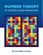Number Theory: In Context and Interactive (Karl-Dieter Crisman)

This is a textbook for an upper-level number theory course, with a clear vision to expose students to the connections to all areas of mathematics, and nearly every concept can be visualized or experimented with using the mathematics software SageMath.

•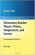Elementary Number Theory: Primes, Congruences, and Secrets

This is a book about prime numbers, congruences, secret messages, and elliptic curves that you can read cover to cover. Many numerical examples are given throughout the book using the SageMath mathematical software.

•An Introduction to the Theory of Numbers (Leo Moser)

This book, which presupposes familiarity only with the most elementary concepts of arithmetic (divisibility properties, greatest common divisor, etc.), is an expanded version of a series of lectures for graduate students on elementary number theory.

•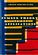Number Theory and Its Applications (Cheon Seoung Ryoo)

Number theory plays a fundamental and important role in mathematics and applied mathematics. This book is based on recent results in all areas related to number theory and its applications.

•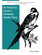An Introductory Course in Elementary Number Theory (Wissam Raji)

This book contains a useful introduction to important topics that need to be addressed in a course in number theory. Proofs of basic theorems are presented in an interesting and comprehensive way that can be read and understood even by non-majors.

•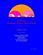Yet Another Introductory Number Theory Textbook (J. A. Poritz)

This introductory number theory textbook has a particular emphasis on connections to cryptology. It broaches the subject of these cryptologic algorithms to develop connections to the topic of number theory.

•Topology of Numbers (Allen Hatcher)

A textbook on elementary number theory from a geometric point of view, as opposed to the usual strictly algebraic approach. A fair amount of the book is devoted to studying Conway's topographs associated to quadratic forms in two variables.

•Magic Squares and Cubes (William Symes Andrews)

This book cover topics such as magic squares, magic cubes, the Franklin squares, magics and Pythagorean numbers, the theory of reversions, magic circles, spheres, and stars, and magic octahedroids, among other things.

•A Computational Introduction to Number Theory and Algebra

This introductory book emphasises algorithms and applications, such as cryptography and error correcting codes, and is accessible to a broad audience. The coverage includes the basics of number theory, abstract algebra and discrete probability theory.

•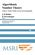Algorithmic Number Theory: Lattices, Curves, and Cryptography

This text provides a comprehensive introduction to algorithmic number theory for beginning graduate students, written by the leading experts in the field. It includes several articles that cover the essential topics in this area.

•A Course in Algebraic Number Theory (Robert B. Ash)

This book explores the general theory of factorization of ideals in Dedekind domains as well as the number field case. Detailed calculations illustrate the use of Kummer's theorem on lifting of prime ideals in extension fields.

•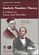Analytic Number Theory: A Tribute to Gauss and Dirichlet

The book begins with a definitive summary of the life and work of Dirichlet and continues with thirteen papers by leading experts on research topics of current interest in number theory that were directly influenced by Gauss and Dirichlet.

•Essays on the Theory of Numbers (Richard Dedekind)

This book contains the two most important essays on the logical foundations of the number system by the famous German mathematician J. W. R. Dedekind. The contents of these essays belong to the foundations of mathematics and our number system.

•Algorithms for Modular Elliptic Curves, 2nd Edition (J. E. Cremona)

This book presents a thorough treatment of many algorithms concerning the arithmetic of elliptic curves, with remarks on computer implementation. An extensive set of tables is provided giving the results of the author's implementation of the algorithms.

•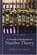A Friendly Introduction to Number Theory (Joseph H. Silverman)

This book is an introductory text designed to entice non-math majors into learning some mathematics, while at the same time teaching them how to think mathematically. The exposition is informal, with a wealth of numerical examples.

Book Categories
 :All CategoriesTop BooksRecent BooksMiscellaneous BooksComputer LanguagesComputer ScienceData Science/DatabasesElectrical EngineeringJava and Java EE (J2EE)Linux and UnixMathematicsMicrosoft and .NETMobile ComputingNetworking and CommunicationsSoftware EngineeringSpecial TopicsWeb Programming
Other Categories